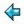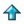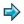### hamming.m

```ham = 1 : foldr1 merge [mult 2 ham, mult 3 ham, mult 5 ham]
where
mult n x = [n*a|a<-x]
merge (a:x) (b:y) = a : merge x y,     if a=b
= a : merge x (b:y), if a<b
= b : merge (a:x) y, if a>b
```

This problem is described by Dijkstra in his book, A Discipline of Programming, and attributed by him to Dr Hamming, of Bell Labs - print in ascending order all numbers of the form 2a . 3b . 5c with a, b, c > 0.

The Miranda solution above is based on a method using communicating processes (see the diagram below): ham is the infinite list of hamming numbers. Note that there is a function called merge in the standard environment, but unlike the one used here it does not remove duplicates from the lists being merged.

PROCESS STRUCTURE OF THE HAMMING PROBLEM